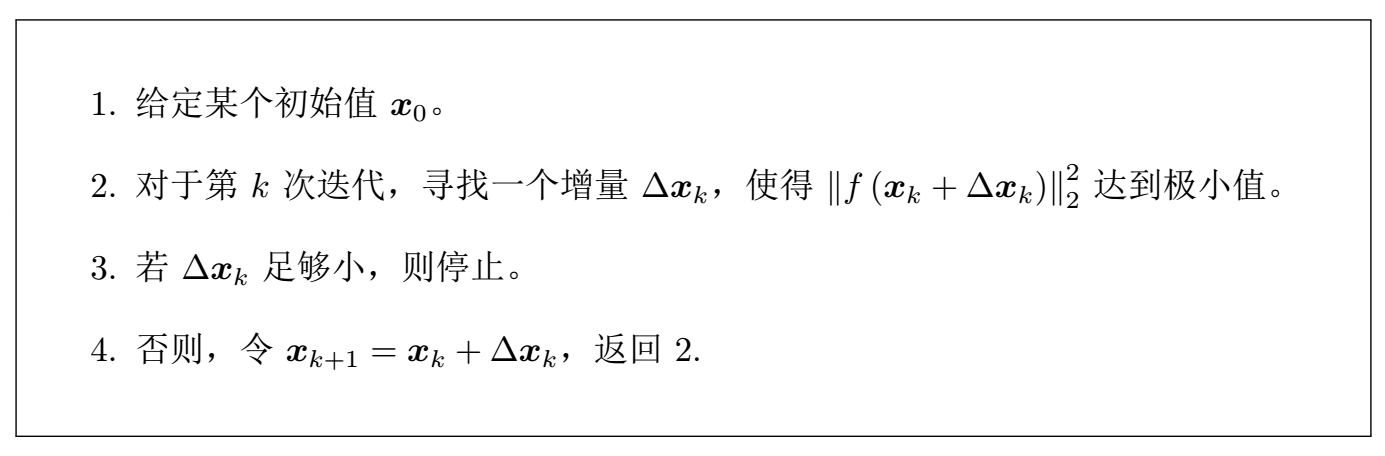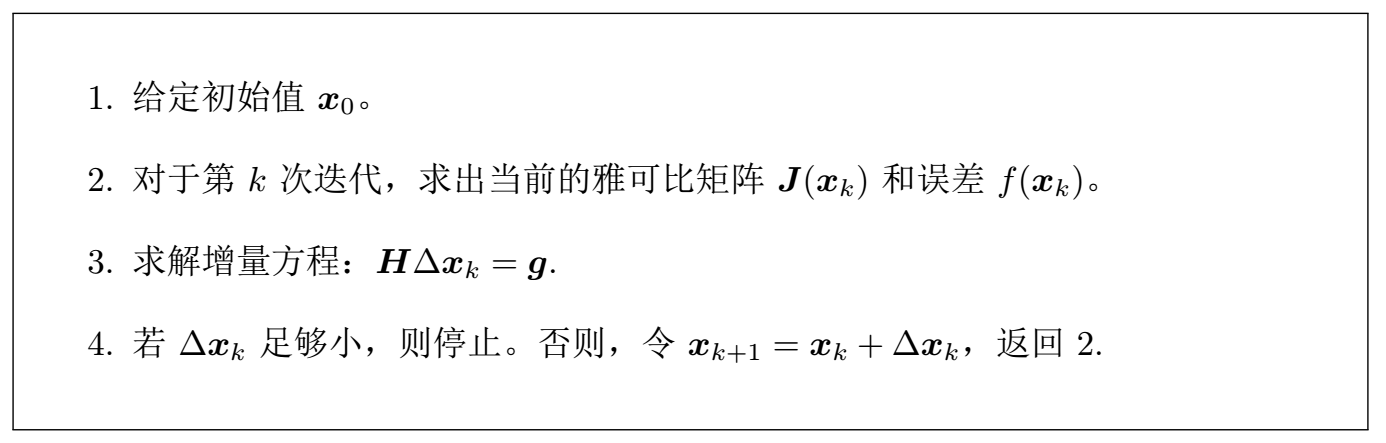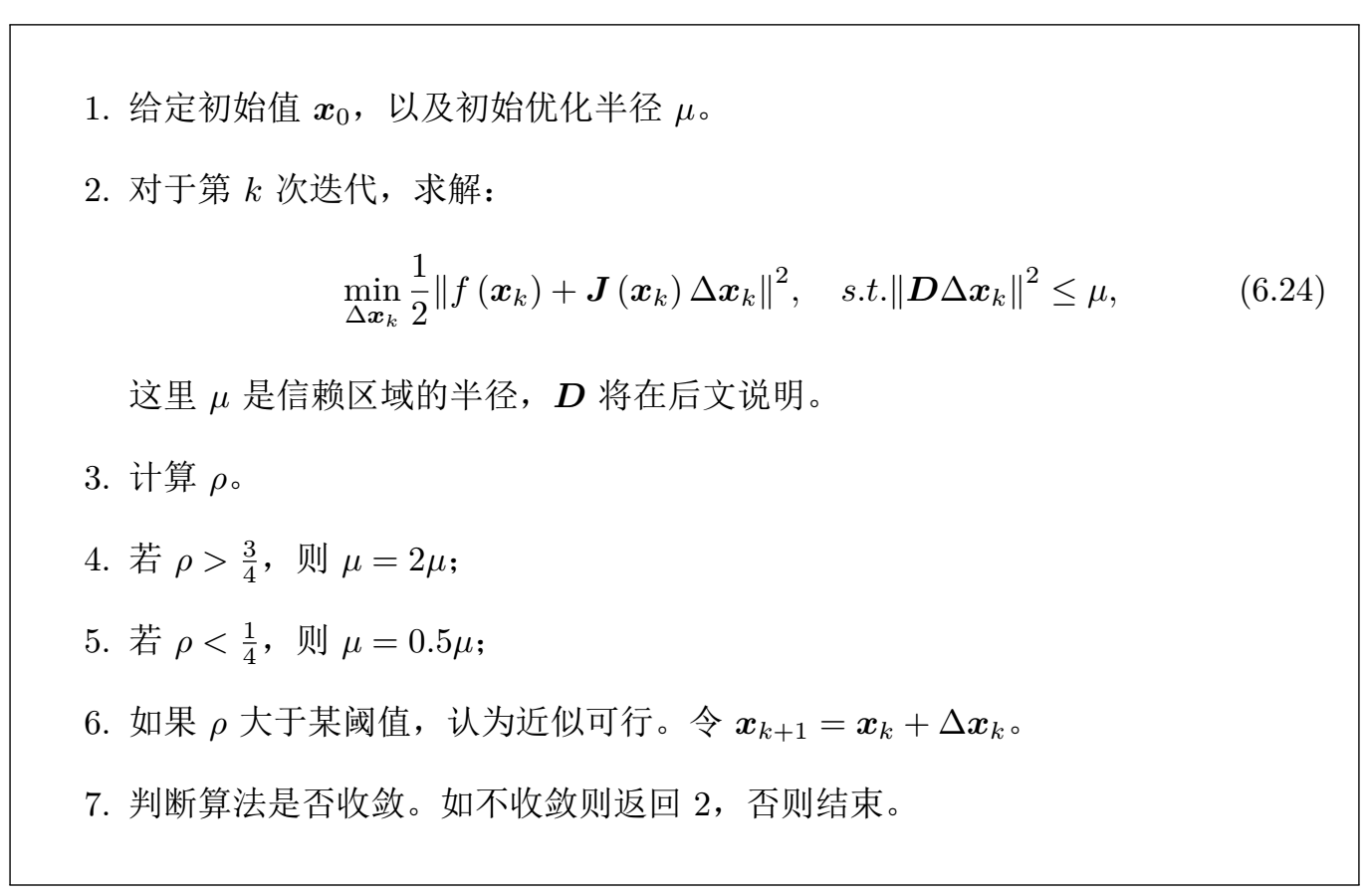# 视觉SLAM十四讲学习笔记——第六章 非线性优化

### 视觉SLAM十四讲学习笔记——第六章 非线性优化# 6.1 状态估计问题

## 6.1.1 最大后验与最大似然

1. 回顾经典 SLAM 模型：2. 在运动和观测方程中，通常假设两个噪声项 $\bm{w_k}, \bm{v_{k,j}}$ 满足零均值的高斯分布3. 状态估计问题：通过带噪声的数据 $\bm{z}$$\bm{u}$ ，推断位姿 $\bm{x}$ 和地图 $\bm{y}$（以及它们的概率分布）。

4. 非线性优化方法比普遍的滤波器方法更有效，成为当前视觉 SLAM 的主流方法。

5. 对机器人状态的估计，就是求已知输入数据 $\bm{u}$ 和观测数据 $\bm{z}$ 的条件下，计算状态 $\bm{x}$条件概率分布$P(\bm{x}|\bm{z}, \bm{u})$，只有一张张的图像时，即只考虑观测方程带来的数据时，相当于估计 $P(\bm{x}|\bm{z})$ 的条件概率分布。

6. 根据贝叶斯法则：7. 贝叶斯法则左侧通常称为后验概率。它右侧的 $P(\bm{z}|\bm{x})$ 称为似然，另一部分 $P(\bm{x})$ 称为先验

8. 直接求后验分布较困难，转而求一个状态最优估计，使得在该状态下，后验概率最大化较为简单：1. 若不知道机器人位姿大概在什么地方，此时就没有了先验。进而可以求解 $\bm{x}$ 的最大似然估计1. 最大似然估计，可以理解成：“在什么样的状态下，最可能产生现在观测到的数据

## 6.1.2 最小二乘的引出

1. 假设噪声项 $\bm{v_k} ∼ N (0, \bm{Q_{k,j}})$，所以观测数据的条件概率为1. 使用最小化负对数的方式，来求一个高斯分布的最大似然。考虑一个任意的高维高斯分布 $\bm{x} ∼ N(\bm{μ}, \bm{Σ})$，它的概率密度函数展开形式为:1. 取负对数1. 第一项与 $\bm{x}$ 无关，可以略去。代入 SLAM 的观测模型，相当于在求：该式等价于最小化噪声项（即误差）的平方（Σ 范数意义下）

2. 对于所有的运动和任意的观测，定义数据与估计值之间的误差并求该误差的平方之和它的最优解等价于状态的最大似然估计。

3. SLAM 中的最小二乘问题具有一些特定的结构：

1. 整个问题的目标函数由许多个误差的（加权的）平方和组成。但每个误差项都是简单的，仅与一两个状态变量有关。每个误差项是一个小规模的约束，把这个误差项的小雅可比矩阵块放到整体的雅可比矩阵中。称每个误差项对应的优化变量为参数块（Parameter Block）
2. 整体误差由很多小型误差项之和组成的问题，其增量方程的求解会具有一定的稀疏性，使得它们在大规模时亦可求解。
3. 使用李代数表示，则该问题是无约束的最小二乘问题
4. 使用了平方形式（二范数）度量误差，它是直观的，相当于欧氏空间中距离的平方

# 6.2 非线性最小二乘

• 对于函数形式简单的最小二乘问题，可以直接使用求导模型。
• 对于不方便直接求解的最小二乘问题，可以用迭代的方式，从一个初始值出发，不断地更新当前的优化变量，使目标函数下降。
具体的步骤为：这让求解导函数为零的问题，变成了一个不断寻找梯度并下降的过程，直到算法收敛

## 6.2.1 一阶和二阶梯度法

1. 将目标函数在 $\bm{x}$ 附近进行泰勒展开：2. 最速下降法：只保留一阶梯度，增量的方向为：即沿着反向梯度方向前进
3. 牛顿法：保留二阶梯度，增量方程为：求右侧等式关于 $\bm{∆x}$ 的导数并令它为零，得到了增量的解：4. 两种方法的问题：
1. 最速下降法过于贪心，容易走出锯齿路线，反而增加了迭代次数。
2. 牛顿法则需要计算目标函数的 $\bm{H}$ 矩阵，这在问题规模较大时非常困难，通常倾向于避免 $\bm{H}$ 的计算

## 6.2.2 Gauss-Newton

1. 思想是将 $f(\bm{x})$ 进行一阶的泰勒展开（注意不是目标函数 $f(\bm{x})^2$）：当前的目标是为了寻找下降矢量 $\bm{∆x}$，使得 $∥f (\bm{x} + \bm{∆x})∥^2$ 达到最小
2. 为了求 $\bm{∆x}$，需要解一个线性的最小二乘问题将上述目标函数对 $\bm{∆x}$ 求导，并令导数为零。这里考虑的是 $\bm{∆x}$ 的导数（而不是 $\bm{x}$
3. 先展开目标函数的平方项：求上式关于 $\bm{∆x}$ 的导数，并令其为零：称它为增量方程，也可以称为高斯牛顿方程 (Gauss Newton equations) 或者正规方程 (Normal equations)。

把左边的系数定义为 $\bm{H}$ ，右边定义为 $\bm{g}$，上式变为：$\bm{H∆x} = \bm{g}$ 。对比牛顿法可见， Gauss-Newton 用 $\bm{J}^T\bm{J}$ 作为牛顿法中二阶 Hessian 矩阵的近似，从而省略了计算 $\bm{H}$ 的过程
4. 求解增量方程是整个优化问题的核心所在，Gauss-Newton 的算法步骤可以写成：5. 高斯-牛顿法的问题
1. 在使用 Gauss Newton 方法时，可能出现 $\bm{J}^T\bm{J}$奇异矩阵或者病态 (illcondition) 的情况，此时增量的稳定性较差，导致算法不收敛。
2. 假设 $\bm{H}$ 非奇异也非病态，如果求出来的步长 $\bm{∆x}$ 太大，也会导致局部近似不够准确，甚至都无法保证它的迭代收敛。

1. 信赖区域方法 (Trust Region Method)：给 $\bm{∆x}$ 添加一个信赖区域（Trust Region），不能让它太大而使得近似不准确。
2. 考虑使用如下公式来判断泰勒近似是否够好如果 $ρ$ 接近于 1，则近似是好的。如果 $ρ$ 太小，说明实际减小的值远少于近似减小的值，则认为近似比较差，需要缩小近似范围。反之，如果 $ρ$ 比较大，则说明实际下降的比预计的更大，我们可以放大近似范围。
3. 改良版的非线性优化框架这里近似范围扩大的倍数和阈值都是经验值，可以替换成别的数值
4. 用 Lagrange 乘子将它转化为一个无约束优化问题：$λ$ 为 Lagrange 乘子

展开后，核心仍是计算增量的线性方程：考虑 $\bm{D} = \bm{I}$，那么相当于求解当参数 $λ$ 比较小时， $\bm{H}$ 占主要地位，这说明二次近似模型在该范围内是比较好的， L-M 方法更接近于 G-N 法。另一方面，当 $λ$ 比较大时， $λ\bm{I}$ 占据主要地位， L-M 更接近于一阶梯度下降法（即最速下降）
5. L-M 的求解方式，可在一定程度上避免线性方程组的系数矩阵的非奇异和病态问题，提供更稳定更准确的增量 $\bm{∆x}$

©️2019 CSDN 皮肤主题: 技术黑板 设计师: CSDN官方博客